• +91 9971497814
• info@interviewmaterial.com

# Triangles EX-6.2 Interview Questions Answers

### Related Subjects

Question 1 : In figure. (i) and (ii), DE || BC. Find EC in (i) and AD in (ii).

Answer 1 :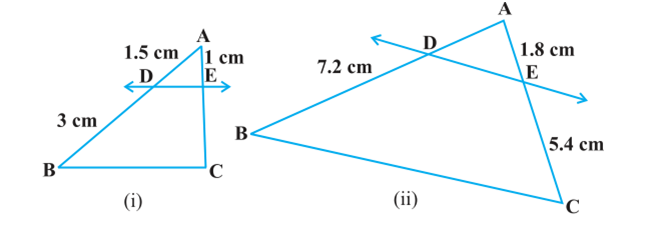Solution

(i) Given, in  ABC, DEBC

AD/DB = AE/EC [Using Basic proportionality theorem]

1.5/3 = 1/EC

EC = 3/1.5

EC = 3×10/15 = 2 cm

Hence, EC = 2 cm.

(ii) Given, in  ABC, DEBC

AD/DB = AE/EC [Using Basic proportionality theorem]

AD = 1.8 ×7.2/5.4 = (18/10)×(72/10)×(10/54) = 24/10

Question 2 : E and F are points on the sides PQ and PR respectively of a ΔPQR. For each of the following cases, state whether EF || QR.

(i) PE = 3.9 cm, EQ = 3 cm, PF = 3.6 cm and FR = 2.4 cm

(ii) PE = 4 cm, QE =4.5 cm, PF = 8 cm and RF = 9 cm
(iii) PQ = 1.28 cm, PR = 2.56 cm, PE = 0.18 cm and PF = 0.63 cm

Solution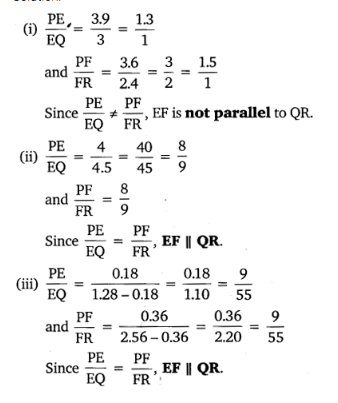Question 3 :

In the figure, if LM || CB and LN || CD, provethat AM/MB = AN/AD

Answer 3 :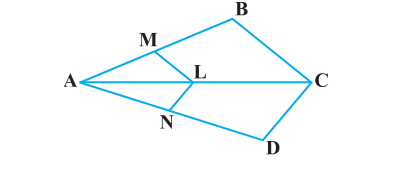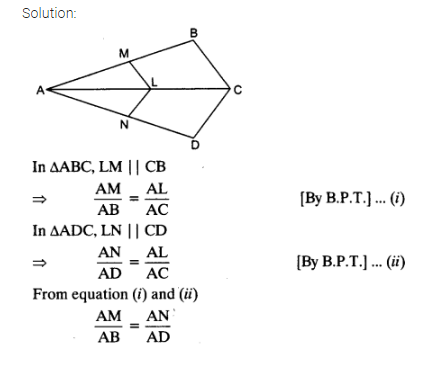Question 4 :

In the figure,DE||AC and DF||AE. Prove that BF/FE = BE/EC

Answer 4 :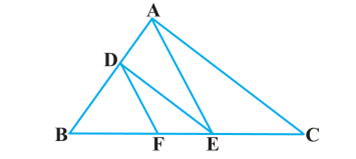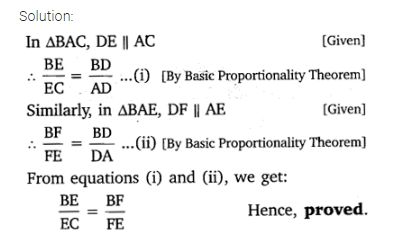Question 5 : In the figure, DE||OQ and DF||OR, show thatEF||QR.

Answer 5 :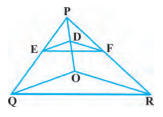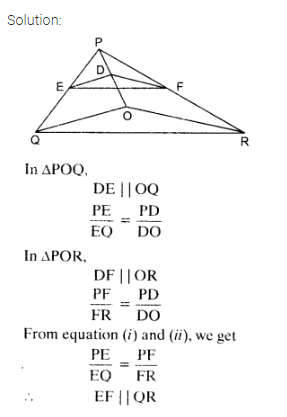Question 6 : In the figure, A, B and C are points on OP, OQand OR respectively such that AB || PQ and AC || PR. Show that BC || QR.

Answer 6 :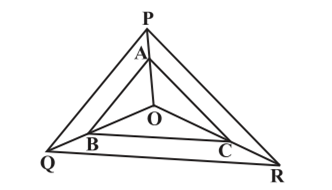Solution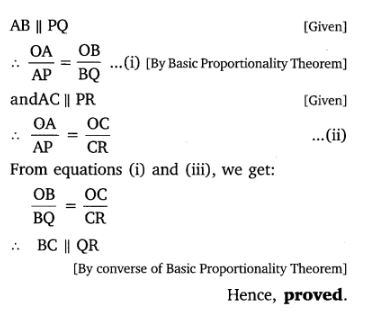Question 7 : Using Basic proportionality theorem, provethat a line drawn through the mid-points of one side of a triangle parallel toanother side bisects the third side. (Recall that you have proved it in ClassIX).

Answer 7 :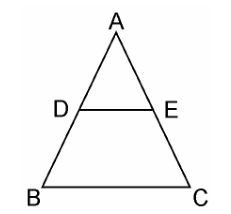Solution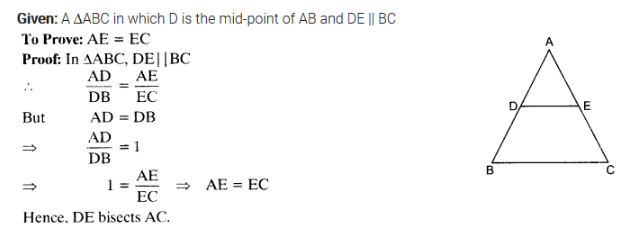Question 8 :

Using Converse ofbasic proportionality theorem, prove that the line joining the mid-points ofany two sides of a triangle is parallel to the third side. (Recall that youhave done it in Class IX).

Answer 8 :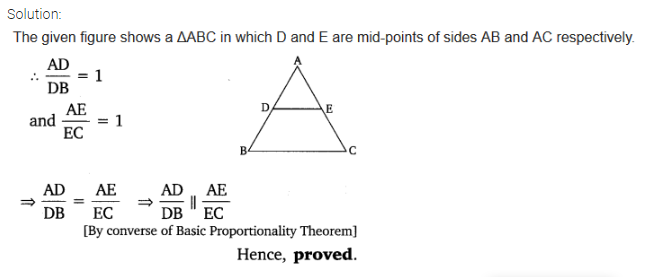Question 9 :

ABCD is a trapeziumin which AB || DC and its diagonals intersect each other at the point O. Showthat AO/BO = CO/DO.

Given, ABCD is a trapezium where AB || DC anddiagonals AC and BD intersect each other at O.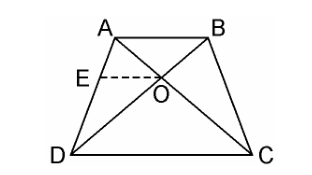We have to prove, AO/BO = CO/DO

From the point O, draw a line EO touching AD at E, in such a way that,

EO || DC || AB

In ΔADC, we have OE || DC

Therefore, By using Basic ProportionalityTheorem

AE/ED = AO/CO ……………..(i)

Now, In ΔABD, OE || AB

Therefore, By using Basic ProportionalityTheorem

DE/EA = DO/BO…………….(ii)

From equation (i) and (ii),we get,

AO/CO = BO/DO

AO/BO = CO/DO

Hence, proved.

Question 10 :

The diagonals of aquadrilateral ABCD intersect each other at the point O such that AO/BO =CO/DO. Show that ABCD is a trapezium.

Given, Quadrilateral ABCD where AC and BDintersects each other at O such that,

AO/BO = CO/DO.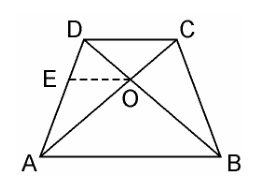We have to prove here, ABCD is a trapezium

From the point O, draw a line EO touching ADat E, in such a way that,

EO || DC || AB

In ΔDAB, EO || AB

Therefore, By using Basic ProportionalityTheorem

DE/EA = DO/OB ……………………(i)

Also, given,

AO/BO = CO/DO

AO/CO = BO/DO

CO/AO = DO/BO

DO/OB = CO/AO …………………………..(ii)

From equation (i) and (ii),we get

DE/EA = CO/AO

Therefore, By using converse of BasicProportionality Theorem,

EO || DC also EO || AB

AB || DC.

Hence, quadrilateral ABCD is a trapezium withAB || CD.

Todays Deals### Triangles EX-6.2 Contributorskrishan

Name:
Email:

# Latest News# 9000 interview questions in different categories